Next: Wave Propagation in Inhomogeneous Up: Traveling Waves Previous: Electromagnetic Waves

# Doppler Effect

Consider a sinusoidal wave of angular frequency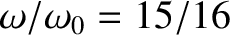and wavenumberthat is propagating in the-direction. We can write represent the wave in terms of a wavefunction of the form(512)

The wavelength and frequency of the wave, as seen by a stationary observer, areand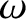, respectively. Consider a second observer moving with uniform speed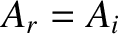in the-direction. What are the wavelength and frequency of the wave seen by the latter observer? Assuming non-relativistic motion, the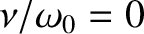-coordinate in the moving observer's frame of reference is given by the standard Gallilean transformation formula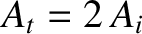(Rindler 1997). Both observers measure the same time. Hence, in the second observer's frame of reference, the wavefunction is written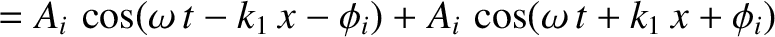(513)

where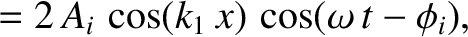(514)

Here, we have replacedby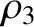in Equation (512). Thus, the moving observer sees a wave possessing the same wavelength ( i.e., the same) but a different frequency (i.e., a different) to that seen by the stationary observer. This phenomenon is known as the Doppler effect. If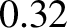is the wave frequency (in hertz) seen by the stationary observer then the wave frequency seen by the moving observer is(515)

where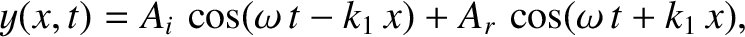is the characteristic wave speed. Thus, an observer moving in the same direction as a wave sees a lower frequency than a stationary observer. On the other hand, an observer moving in the opposite direction to a wave sees a higher frequency than a stationary observer. Hence, the general Doppler shift formula (for a moving observer and a stationary wave source) is(516)

where the upper/lower signs correspond to the observer moving in the same/opposite direction to the wave.

Consider a stationary observer measuring a wave emitted by a source that is moving towards the observer with speed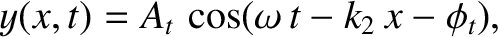. Letbe the characteristic propagation speed of the wave. Consider two neighboring wave crests emitted by the source. Suppose that the first is emitted at time, and the second at time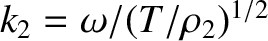, whereis the wave period in the frame of reference of the source. At time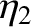, the first wave crest has traveled a distancetowards the observer, whereas the second wave crest has traveled a distance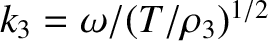(measured from the position of the source at). Here, we have taken into account the fact that the source is a distance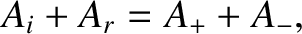closer to the observer when the second wave crest is emitted. The effective wavelength,, seen by the observer is the distance between neighboring wave crests. Hence,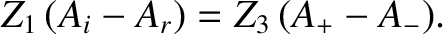(517)

Because, the effective frequency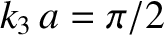seen by the observer is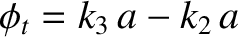(518)

whereis the wave frequency in the frame of reference of the source. We conclude that if the source is moving towards the observer then the wave frequency is shifted upwards. Likewise, if the source is moving away from the observer then the frequency is shifted downwards. This manifestation of the Doppler effect is familiar from everyday experience. When an ambulance passes us on the street, its siren has a higher pitch (i.e., a high frequency) when it is coming towards us than when it is moving away from us. In fact, the oscillation frequency of the siren never changes. It is the Doppler shift induced by the motion of the siren with respect to a stationary listener that causes the frequency change.

The general formula for the shift in wave frequency induced by relative motion of an observer and a source is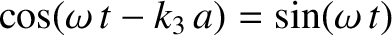(519)

whereis the speed of the observer, andis the speed of the source (both measured relative to the wave medium). The upper/lower signs correspond to relative motion by which the observer and the source move apart/together. If the observer and source are not moving directly toward or directly away from one another then the quantitiesandin the above formulae correspond to the components of the observer and source velocities, respectively, along the straight-line that instantaneously joins them.

An important proviso to the previous formula is that it is strictly classical, and only holds for non-relativistic motion (i.e.,,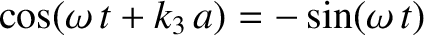, where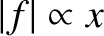is the velocity of light in vacuum.) In fact, when applied to light propagation in a vacuum, the formula is only accurate up to first-order in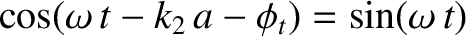and(Rindler 1997). In other words, for light propagation the previous equation reduces to(520)

where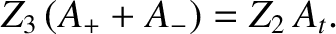is the relative radial velocity of the source with respect to the observer (being positive when the source and observer are moving apart, and vice versa).

Probably the most well-known use of the Doppler effect in everyday life is in police speed traps. In such a trap, a policeman fires radar waves (i.e., electromagnetic waves of centimeter wavelength) of fixed frequency at an oncoming car. These waves reflect off the car, which effectively becomes a moving source. Hence, by measuring the frequency increase of the reflected waves, the policeman can determine the car's speed.Next: Wave Propagation in Inhomogeneous Up: Traveling Waves Previous: Electromagnetic Waves
Richard Fitzpatrick 2013-04-08